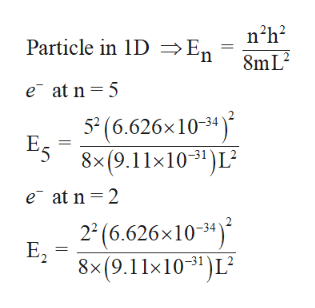Consider an electron in a nanowire (approximated by a one-adimensional box) that goes from the state with n=5 to the state with n = 2. In doing so, it emits a photon with a frequency of 6.0 x 10^14 s^-1. Find the length of the wire in nm.

Question

Consider an electron in a nanowire (approximated by a one-adimensional box) that goes from the state with n=5 to the state with n = 2. In doing so, it emits a photon with a frequency of 6.0 x 10^14 s^-1. Find the length of the wire in nm.

Step 1

The energy equation for electron pres...help_outlineImage Transcriptionclosenh2 Particle in IDE, 8mL2 e at n 5 5(6.626x10-34) 58x(9.11x103")L2 e at n 2 2 2 (6.626x103) E2 8x(9.11x1031)L2 | fullscreen

Want to see the full answer?

See Solution

Want to see this answer and more?

Our solutions are written by experts, many with advanced degrees, and available 24/7

See Solution
Tagged in

Chemistry